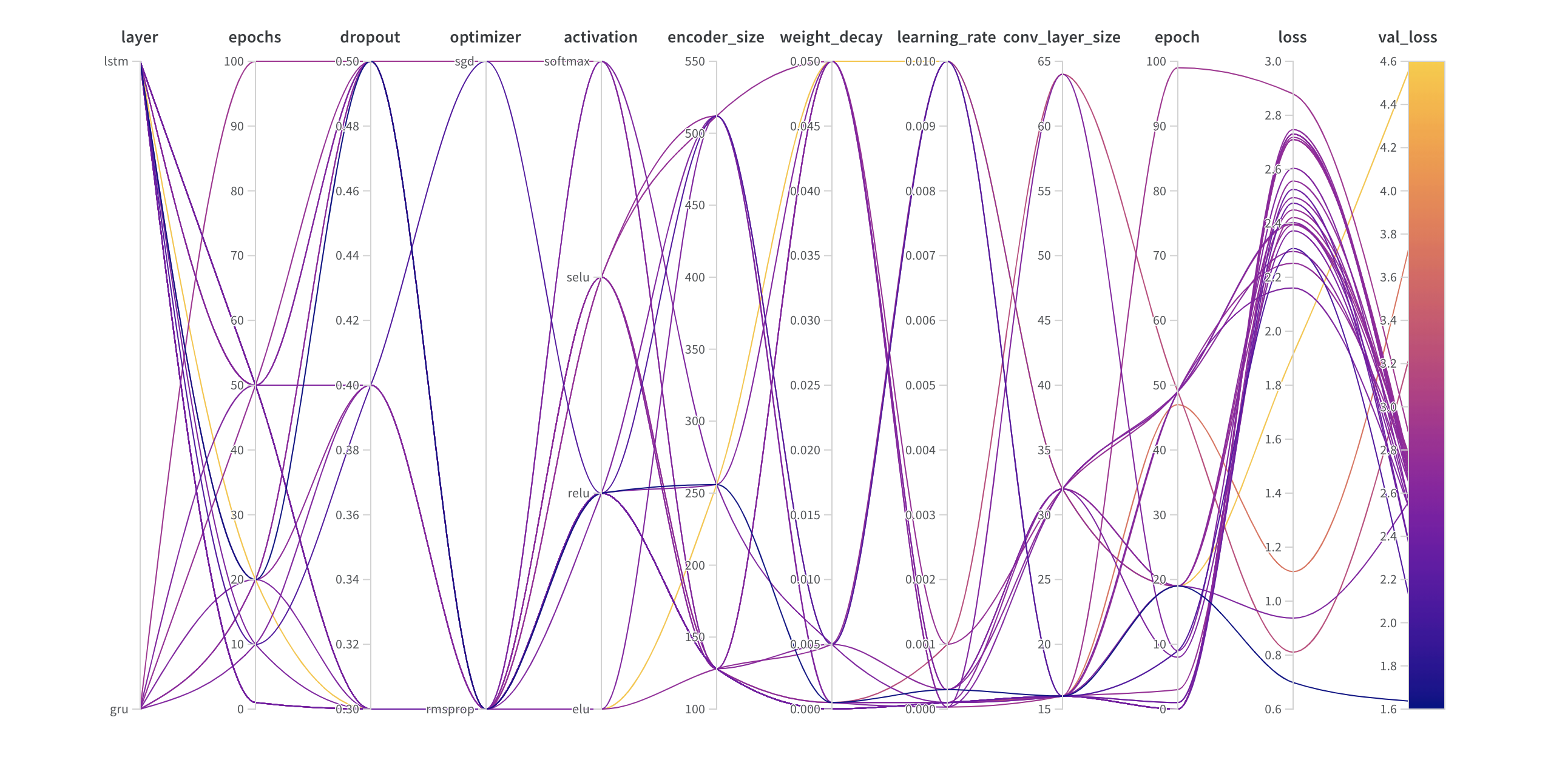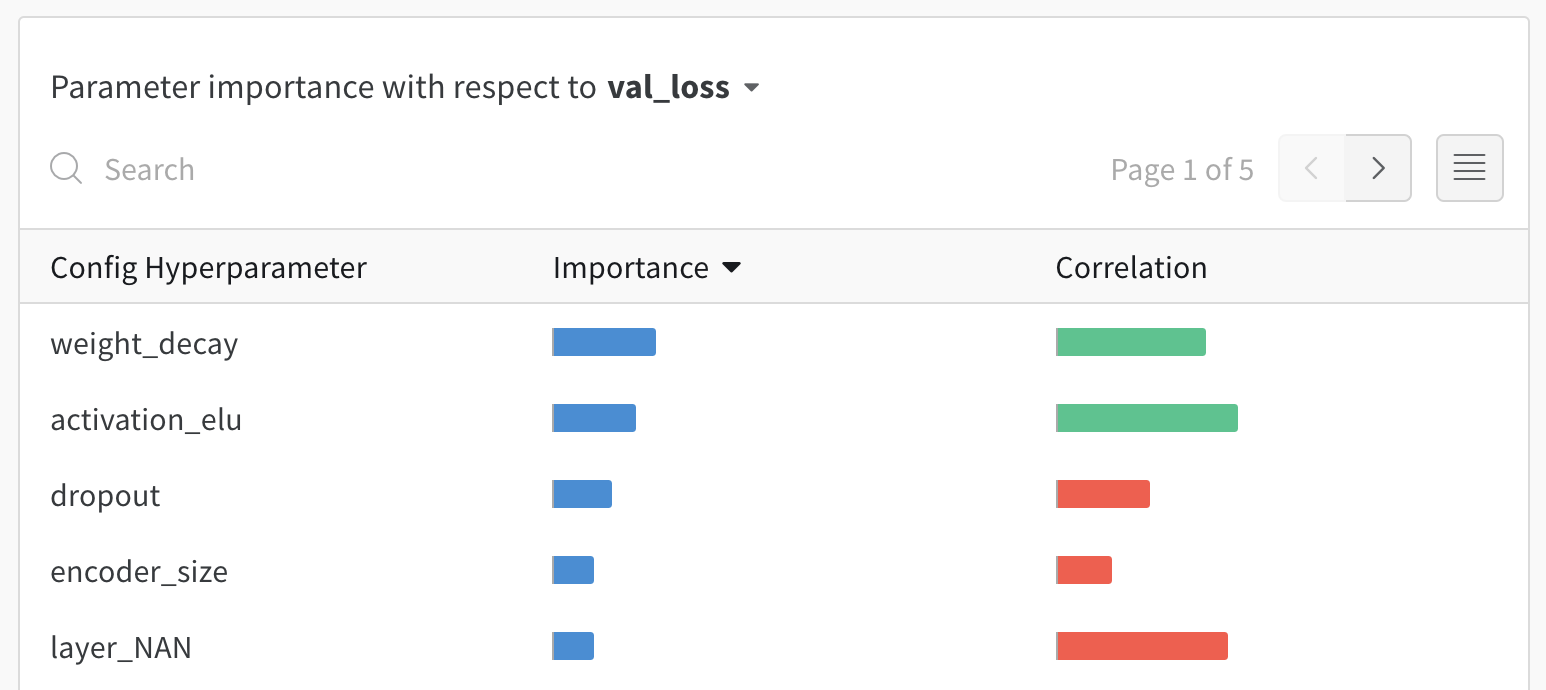# Tune hyperparameters

Try in a Colab Notebook here →

Searching through high dimensional hyperparameter spaces to find the most performant model can get unwieldy very fast. Hyperparameter sweeps provide an organized and efficient way to conduct a battle royale of models and pick the most accurate model. They enable this by automatically searching through combinations of hyperparameter values (e.g. learning rate, batch size, number of hidden layers, optimizer type) to find the most optimal values.

In this tutorial, we'll see how you can run sophisticated hyperparameter sweeps in 3 easy steps using Weights & Biases.

### Follow along with a video tutorial!​# 🚀 Setup

Start out by installing the experiment tracking library and setting up your free W&B account:

1. Install with `!pip install`
2. `import` the library into Python
3. `.login()` so you can log metrics to your projects

If you've never used Weights & Biases before, the call to `login` will give you a link to sign up for an account. W&B is free to use for personal and academic projects!

``!pip install wandb -Uq``
``import wandbwandb.login()``

# Step 1️⃣. Define the Sweep

Fundamentally, a Sweep combines a strategy for trying out a bunch of hyperparameter values with the code that evalutes them. You just need to define your strategy in the form of a configuration.

When you're setting up a Sweep in a notebook like this, that config object is a nested dictionary. When you run a Sweep via the command line, the config object is a YAML file.

Let's walk through the definition of a Sweep config together. We'll do it slowly, so we get a chance to explain each component. In a typical Sweep pipeline, this step would be done in a single assignment.

### 👈 Pick a `method`​

The first thing we need to define is the `method` for choosing new parameter values.

We provide the following search `methods`:

• `grid` Search – Iterate over every combination of hyperparameter values. Very effective, but can be computationally costly.
• `random` Search – Select each new combination at random according to provided `distribution`s. Surprisingly effective!
• `bayes`ian Search – Create a probabilistic model of metric score as a function of the hyperparameters, and choose parameters with high probability of improving the metric. Works well for small numbers of continuous parameters but scales poorly.

We'll stick with `random`.

``sweep_config = {    'method': 'random'    }``

For `bayes`ian Sweeps, you also need to tell us a bit about your `metric`. We need to know its `name`, so we can find it in the model outputs and we need to know whether your `goal` is to `minimize` it (e.g. if it's the squared error) or to `maximize` it (e.g. if it's the accuracy).

``metric = {    'name': 'loss',    'goal': 'minimize'       }sweep_config['metric'] = metric``

If you're not running a `bayes`ian Sweep, you don't have to, but it's not a bad idea to include this in your `sweep_config` anyway, in case you change your mind later. It's also good reproducibility practice to keep note of things like this, in case you, or someone else, come back to your Sweep in 6 months or 6 years and don't know whether `val_G_batch` is supposed to be high or low.

### 📃 Name the hyper`parameters`​

Once you've picked a `method` to try out new values of the hyperparameters, you need to define what those `parameters` are.

Most of the time, this step is straightforward: you just give the `parameter` a name and specify a list of legal `values` of the parameter.

For example, when we choose the `optimizer` for our network, there's only a finite number of options. Here we stick with the two most popular choices, `adam` and `sgd`. Even for hyperparameters that have potentially infinite options, it usually only makes sense to try out a few select `values`, as we do here with the hidden `layer_size` and `dropout`.

``parameters_dict = {    'optimizer': {        'values': ['adam', 'sgd']        },    'fc_layer_size': {        'values': [128, 256, 512]        },    'dropout': {          'values': [0.3, 0.4, 0.5]        },    }sweep_config['parameters'] = parameters_dict``

It's often the case that there are hyperparameters that we don't want to vary in this Sweep, but which we still want to set in our `sweep_config`.

In that case, we just set the `value` directly:

``parameters_dict.update({    'epochs': {        'value': 1}    })``

For a `grid` search, that's all you ever need.

For a `random` search, all the `values` of a parameter are equally likely to be chosen on a given run.

If that just won't do, you can instead specify a named `distribution`, plus its parameters, like the mean `mu` and standard deviation `sigma` of a `normal` distribution.

See more on how to set the distributions of your random variables here.

``parameters_dict.update({    'learning_rate': {        # a flat distribution between 0 and 0.1        'distribution': 'uniform',        'min': 0,        'max': 0.1      },    'batch_size': {        # integers between 32 and 256        # with evenly-distributed logarithms         'distribution': 'q_log_uniform_values',        'q': 8,        'min': 32,        'max': 256,      }    })``

When we're finished, `sweep_config` is a nested dictionary that specifies exactly which `parameters` we're interested in trying and what `method` we're going to use to try them.

``import pprintpprint.pprint(sweep_config)``

But that's not all of the configuration options!

For example, we also offer the option to `early_terminate` your runs with the HyperBand scheduling algorithm. See more here.

You can find a list of all configuration options here and a big collection of examples in YAML format here.

# Step 2️⃣. Initialize the Sweep

Once you've defined the search strategy, it's time to set up something to implement it.

The clockwork taskmaster in charge of our Sweep is known as the Sweep Controller. As each run completes, it will issue a new set of instructions describing a new run to execute. These instructions are picked up by agents who actually perform the runs.

In a typical Sweep, the Controller lives on our machine, while the agents who complete runs live on your machine(s), like in the diagram below. This division of labor makes it super easy to scale up Sweeps by just adding more machines to run agents!We can wind up a Sweep Controller by calling `wandb.sweep` with the appropriate `sweep_config` and `project` name.

This function returns a `sweep_id` that we will later user to assign agents to this Controller.

Side Note: on the command line, this function is replaced with

``wandb sweep config.yaml``

``sweep_id = wandb.sweep(sweep_config, project="pytorch-sweeps-demo")``

# Step 3️⃣. Run the Sweep agent

### 💻 Define Your Training Procedure​

Before we can actually execute the sweep, we need to define the training procedure that uses those values.

In the functions below, we define a simple fully-connected neural network in PyTorch, and add the following `wandb` tools to log model metrics, visualize performance and output and track our experiments:

• `wandb.init()` – Initialize a new W&B Run. Each Run is a single execution of the training function.
• `wandb.config` – Save all your hyperparameters in a configuration object so they can be logged. Read more about how to use `wandb.config` here.
• `wandb.log()` – log model behavior to W&B. Here, we just log the performance; see this Colab for all the other rich media that can be logged with `wandb.log`.

For more details on instrumenting W&B with PyTorch, see this Colab.

``import torchimport torch.optim as optimimport torch.nn.functional as Fimport torch.nn as nnfrom torchvision import datasets, transformsdevice = torch.device("cuda" if torch.cuda.is_available() else "cpu")def train(config=None):    # Initialize a new wandb run    with wandb.init(config=config):        # If called by wandb.agent, as below,        # this config will be set by Sweep Controller        config = wandb.config        loader = build_dataset(config.batch_size)        network = build_network(config.fc_layer_size, config.dropout)        optimizer = build_optimizer(network, config.optimizer, config.learning_rate)        for epoch in range(config.epochs):            avg_loss = train_epoch(network, loader, optimizer)            wandb.log({"loss": avg_loss, "epoch": epoch})           ``

This cell defines the four pieces of our training procedure: `build_dataset`, `build_network`, `build_optimizer`, and `train_epoch`.

All of these are a standard part of a basic PyTorch pipeline, and their implementation is unaffected by the use of W&B, so we won't comment on them.

``def build_dataset(batch_size):       transform = transforms.Compose(        [transforms.ToTensor(),         transforms.Normalize((0.1307,), (0.3081,))])    # download MNIST training dataset    dataset = datasets.MNIST(".", train=True, download=True,                             transform=transform)    sub_dataset = torch.utils.data.Subset(        dataset, indices=range(0, len(dataset), 5))    loader = torch.utils.data.DataLoader(sub_dataset, batch_size=batch_size)    return loaderdef build_network(fc_layer_size, dropout):    network = nn.Sequential(  # fully-connected, single hidden layer        nn.Flatten(),        nn.Linear(784, fc_layer_size), nn.ReLU(),        nn.Dropout(dropout),        nn.Linear(fc_layer_size, 10),        nn.LogSoftmax(dim=1))    return network.to(device)        def build_optimizer(network, optimizer, learning_rate):    if optimizer == "sgd":        optimizer = optim.SGD(network.parameters(),                              lr=learning_rate, momentum=0.9)    elif optimizer == "adam":        optimizer = optim.Adam(network.parameters(),                               lr=learning_rate)    return optimizerdef train_epoch(network, loader, optimizer):    cumu_loss = 0    for _, (data, target) in enumerate(loader):        data, target = data.to(device), target.to(device)        optimizer.zero_grad()        # ➡ Forward pass        loss = F.nll_loss(network(data), target)        cumu_loss += loss.item()        # ⬅ Backward pass + weight update        loss.backward()        optimizer.step()        wandb.log({"batch loss": loss.item()})    return cumu_loss / len(loader)``

Now, we're ready to start sweeping! 🧹🧹🧹

Sweep Controllers, like the one we made by running `wandb.sweep`, sit waiting for someone to ask them for a `config` to try out.

That someone is an `agent`, and they are created with `wandb.agent`. To get going, the agent just needs to know

1. which Sweep it's a part of (`sweep_id`)
2. which function it's supposed to run (here, `train`)
3. (optionally) how many configs to ask the Controller for (`count`)

FYI, you can start multiple `agent`s with the same `sweep_id` on different compute resources, and the Controller will ensure that they work together according to the strategy laid out in the `sweep_config`. This makes it trivially easy to scale your Sweeps across as many nodes as you can get ahold of!

Side Note: on the command line, this function is replaced with

``wandb agent sweep_id``

The cell below will launch an `agent` that runs `train` 5 times, usingly the randomly-generated hyperparameter values returned by the Sweep Controller. Execution takes under 5 minutes.

``wandb.agent(sweep_id, train, count=5)``

# 👀 Visualize Sweep Results

## 🔀 Parallel Coordinates Plot​

This plot maps hyperparameter values to model metrics. It’s useful for honing in on combinations of hyperparameters that led to the best model performance.## 📊 Hyperparameter Importance Plot​

The hyperparameter importance plot surfaces which hyperparameters were the best predictors of your metrics. We report feature importance (from a random forest model) and correlation (implicitly a linear model).These visualizations can help you save both time and resources running expensive hyperparameter optimizations by honing in on the parameters (and value ranges) that are the most important, and thereby worthy of further exploration.

# 🧤 Get your hands dirty with sweeps

We created a simple training script and a few flavors of sweep configs for you to play with. We highly encourage you to give these a try.

That repo also has examples to help you try more advanced sweep features like Bayesian Hyperband, and Hyperopt.

# What's next?

In the next tutorial, you will learn how to manage model weights & dataset versions using W&B Artifacts: